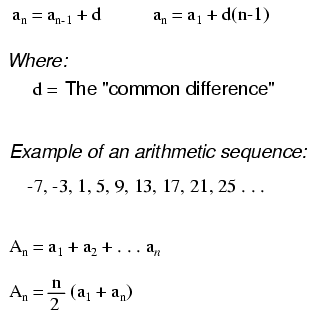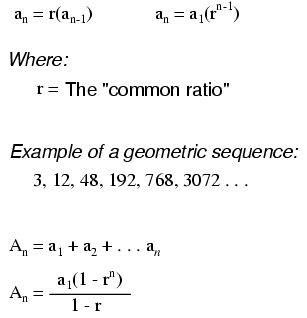# Learning Electronics

Learn to build electronic circuits

# Sequences

### Arithmetic sequences

An arithmetic sequence is a series of numbers obtained by adding (or subtracting) the same value with each step. A child's counting sequence (1, 2, 3, 4, . . .) is a simple arithmetic sequence, where the common difference is 1: that is, each adjacent number in the sequence differs by a value of one. An arithmetic sequence counting only even numbers (2, 4, 6, 8, . . .) or only odd numbers (1, 3, 5, 7, 9, . . .) would have a common difference of 2.

In the standard notation of sequences, a lower-case letter "a" represents an element (a single number) in the sequence. The term "an" refers to the element at the nth step in the sequence. For example, "a3" in an even-counting (common difference = 2) arithmetic sequence starting at 2 would be the number 6, "a" representing 4 and "a1" representing the starting point of the sequence (given in this example as 2).

A capital letter "A" represents the sum of an arithmetic sequence. For instance, in the same even-counting sequence starting at 2, A4 is equal to the sum of all elements from a1 through a4, which of course would be 2 + 4 + 6 + 8, or 20.### Geometric sequences

A geometric sequence, on the other hand, is a series of numbers obtained by multiplying (or dividing) by the same value with each step. A binary place-weight sequence (1, 2, 4, 8, 16, 32, 64, . . .) is a simple geometric sequence, where the common ratio is 2: that is, each adjacent number in the sequence differs by a factor of two.